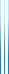# Courses

236646 Boolean Function Analysis Winter 2015/2016

During the 2015/2016 winter semester, I gave a course on Analysis of Boolean functions, based on Ryan O’Donnell’s recent monograph.

I also prepared lecture notes.

First assignment, due 25 November 2015. Assignment and solutions.

Second assignment, due 16 December 2015. Assignment and solutions.

Third assignment is to give a two-hour long seminar:

• On subsets of finite abelian groups containing no 3-term arithmetic progressions by Roy Meshulam (Itay Hazan).
This paper proves an analog of Roth’s theorem for groups such as $\mathbb{Z}_3^n$ (Roth’s theorem states that every “large enough” subset of the integers contains infinitely many three-term arithmetic progressions). Other possible sources are notes by Ryan O’Donnell. You might need also to explain how Fourier analysis works on $\mathbb{Z}_3^n$ (see Chapter 10 in my lecture notes).
• Reed–Muller codes achieve capacity on erasure channels by Santhosh Kumar and Henry D. Pfister (Yuval Dagan).
This paper shows that Reed–Muller codes, a type of error-correcting codes, can be used to transmit information at the optimal rate through a channel in which some of the symbols are erased (the receiver knows the positions of the erased symbols). This is an application of a deep sharp threshold theorem of Bourgain and Kalai.
• A simple reduction from a biased measure on the discrete cube to the uniform measure by Nathan Keller (Ori Sberlo).
This paper gives a method which enables generalizing results from the uniform distribution over $\{\pm1\}^n$ to the biased measure $\mu_p$. As applications, the author proves a biased version of the Bonami–Beckner hypercontractive inequality, give only its standard version.
• Tight bounds on the Fourier spectrum of $\mathsf{AC^0}$ by Avishay Tal (Sa’ar Zehavi).
The paper is composed of two independent parts. In the first, the author strengthens the classical result of Linial, Mansour and Nisan on the tails of Boolean functions computed by $\mathsf{AC^0}$ circuits.
In the second, he shows that bounds of this sort are equivalent to several other spectral bounds appearing in the literature.
As corollaries of both parts, the author improves and simplifies proofs of many classical results on $\mathsf{AC^0}$ circuits.

## OTHER SEMESTERS

• 236646 Boolean Function Analysis Spring 2019